# Limits on the AP Calculus Exam: A Review

Limits are one of the first topics we need to master in our calculus courses.  There are many times when a function does not take on a value at a specific point.  For instance, let’s say we take the function f(x) = sin(x)/x.  What is the value of this function at x = 0?  Clearly, we cannot divide by 0, so there is a hole in this graphthere is no value for the function at x = 0.  However, if we look at the graph below, we see that the function gets closer and closer to 1 as x gets closer and closer to 0.This is the idea of a limit.  Even if we do not have the exact value of the function at a certain value of x, we might still know what value the function gets closer and closer to as we get closer and closer to a specific x.

In other words, a limit is the value that a function approaches as an input approaches a specific value.

## AP Calculus Exam Review: Notation of Limits

The AP exam writes limits in the following manner: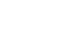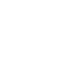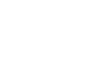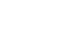The first example above is read as the ‘the limit of f(x), as x approaches n’.  If we look at the three examples, we’ll notice that sometimes you’ll see a + or a – in the limit.  If we see a +, this means ‘from the right’ or ‘from the positive.’  A negative means ‘from the left’ or ‘from the negative.’  We will discuss this in more depth below.

## How to Calculate Limits

There are several scenarios that the AP Exam might ask about in regards to determining limits.  If the question exists on the calculator section, a graphing calculator can be used to quickly solve limits.  Simply graph the function, and set the window to the domain in which we are looking, and see what the graph is doing.

For example, let us take the graph below:This is the graph of f(x) = 1/x.  There is no value for this function as x=0 (remember, we can never divide by 0 in any equation).  However, the graph shows that as we approach x= 0 from the right, the graph approaches positive infinity.  From the left, negative infinity.  The ‘trace’ function on many graphing calculators is useful in figuring out the value a graph is approaching.

However, most limit questions will not involve the calculator, or can be more quickly solved analytically.

## 3 Most Common Limit Scenarios

There are a few scenarios that the AP exam might ask.  Almost all limit questions will involve a fraction: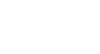### Scenario #1

In the first scenario, we will be asked to evaluate a limit where the denominator evaluates to 0, but the numerator evaluates to a non-zero number.  In such a case, the answer is always infinity or negative infinity (can you see why this is the case?).

To determine which, carefully look at the numerator and the denominator separately.  The numerator will always be positive or negative.  The denominator will be 0, but look at which way we are approaching from.  If from the positive, pick a number just to the right of the limit (in this case, we can take 3.1).  If it asked from the negative, we could take a number just to the left.  Does this now evaluate to positive or negative?  3.1-3 is positive, so we have a positive numerator and denominator, and our limit evaluates to positive infinity.  If our numerator and denominator are the same sign, our limit is positive infinity; Different signs, negative infinity.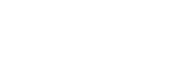### Scenario #2

The second scenario is if there is no fraction, or the denominator does not evaluate to 0.  Chances are, in this case, the exam is asking us to see what happens as the limit approaches infinity. In such a case, we just need to ask ourselves is the limit getting bigger and bigger forever, or is it approaching a certain number?  In the first example above, as x gets bigger, the function gets bigger, so the limit approaches infinity.  In the second, the function gets closer and closer to 2.  If we’re ever not sure, just plug in a very large number and try to get a sense of what the function is doing.

### Scenario #3

The third scenario involves limits where both the numerator and denominator both approach 0 or infinity.In the above example, if we plug in 5 to the function, we get 0/0, which doesn’t help us.  There are two common ways of solving these types of questions.

First, we can sometimes factor the equations.  In the above example we get:We can see that the x-5 term in each equation cancels out, leaving us with (x+3)/(x+2).  Plug in 5, and we get (5+3)/(5+2) = 8/7, the solution to our question.

This won’t always be the case, and there is a great shortcut anytime we have a limit that evaluates to 0/0 or ±infinity/±infinity: L’Hospital’s Rule.  If we take the derivative of the numerator and denominator separately, we can evaluate the limit.In our above example:Evaluating, we get (10-2)/(10-3) or 8/7, the same answer we got from factoring.  This is often a quicker way of solving than factoring.  In addition, it works on equations where factoring does not help.  For instance:We will have more posts soon with tricks and practice problems regarding limits.  Limits are a great place to start studying as they are an important foundation for much of your calculus course.

#### Improve your SAT or ACT score, guaranteed. Start your 1 Week Free Trial of Magoosh SAT Prep or your 1 Week Free Trial of Magoosh ACT Prep today!## Author

•Zachary is a former mechanical engineer and current high school physics, math, and computer science teacher. He graduated from McGill University in 2011 and spent time in the automotive industry in Detroit before moving into education. He has been teaching and tutoring for the past five years, but you can also find him adventuring, reading, rock climbing, and traveling whenever the opportunity arises.